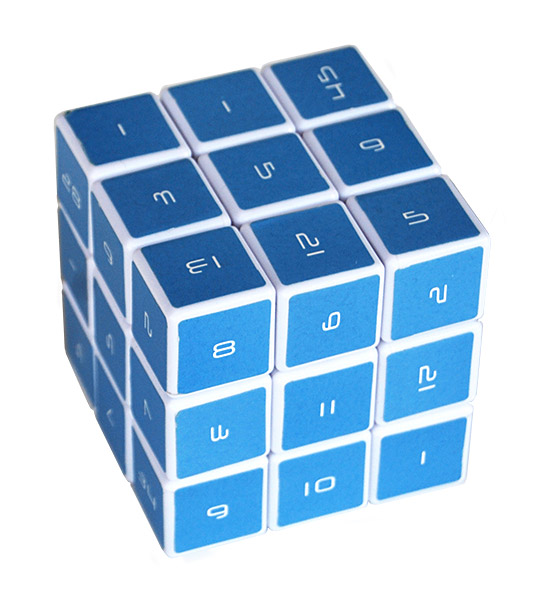# The Magic Cube: Mathematic 3D Logic PuzzleMath geeks, this one is for you. Besides, I don’t have the brains to figure this cube out. The Magic Cube: Mathematic 3D Logic Puzzle is a puzzle cube surrounded by special numbers and each face has a different series of numbers. Fibonacci, digits of Pi, and more.

Like I said, real braniac stuff. Just do the usual thing that you do when solving a Rubik’s cube, but also apply your math skills to putting all like numbers together as well.

The Magic Cube: Mathematic 3D Logic Puzzle

• The number series on each of the six faces of the Magic Cube represent a selection of the most famous series or numerical constants in mathematical number theory.
• Number Series Roll Call: Triangle Series, Prime Number Series, Digits of Pi, Magic Square, Fibonacci Series, and Digits of E.
• To solve the Magic Cube, all the faces must show the six mathematical sequences, and all of the numbers must have the same orientation.
• Dimensions: approx. 2.75″ cubed.

The Magic Cube: Mathematic 3D Logic Puzzle is \$19.99 from ThinkGeek.com.